# Angle + addition - math problems

#### Number of problems found: 23

• Calculate 20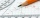Calculate the sum of 1/5 of a right angle and 3/4 of a right angle and 3/4 of a straight angle
• Supplementary angles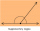(a + 30)° and (2a)° are the measure of two supplementary angles. Find the value of 'a'.
• A straight angle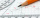A straight angle measures s, so you can subtract or add 85 from What are the measure of their angles?
• The sum 11The sum of the measures of two angles is 89°. One angle has a measure of 64°. What is the measure in degrees of the second angle?
• The two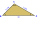The two angles of a triangle are 78° and 82°. So what is the measure of the remaining third angle?
• PetraPetra likes to bathe in a bathtub with a water temperature of 38 degrees. She turned on the hot water set at 70 degrees and left for a moment. Before she returned, 40 liters of water flowed into the tub. How much water with a temperature of 22 degrees mus
• Mass point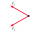Two equal forces of 30 Newtons act on a mass point. Find the magnitude of the resultant force if these forces form an angle of 42°.
• Ground levelThe temperature at ground level in Helsinki is 20°C lower than in London. The temperature in a basement flat in Helsinki is 5°C below the temperature at ground level. If it is -29°c in the basement, what is the temperature in London?
• Angles of a hexagon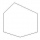Find the interior angles of a hexagon if the sizes of the angles form an arithmetic sequence, and the smallest angle is 70°.
• Trapezoid 25Trapezoid PART with AR||PT has (angle P=x) and (angle A=2x) . In addition, PA = AR = RT = s. Find the length of the median of Trapezoid PART in terms of s.
• Six-sided polygon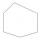In a six-sided polygon. The first two angles are equal, the third angle is twice (the equal angles), two other angles are trice the equal angle, while the last angle is a right angle. Find the value of each angle.
• Resultant forceCalculate mathematically and graphically the resultant of three forces with a common center if: F1 = 50 kN α1 = 30° F2 = 40 kN α2 = 45° F3 = 40 kN α3 = 25°
• Octahedron - sumOn each wall of a regular octahedron is written one of the numbers 1, 2, 3, 4, 5, 6, 7 and 8, wherein on different sides are different numbers. For each wall John make the sum of the numbers written of three adjacent walls. Thus got eight sums, which also
• Three vectorsThe three forces whose amplitudes are in ratio 9:10:17 act in the plane at one point to balance. Determine the angles of each two forces.
• ForcesForces with magnitudes F1 = 42N and F2 = 35N act at a common point and make an angle of 77°12'. How big is their resultant?
• BearingA plane flew 50 km on a bearing 63°20' and the flew on a bearing 153°20' for 140km. Find the distance between the starting point and the ending point.
• TrianglesFind out whether given sizes of the angles can be interior angles of a triangle: a) 23°10',84°30',72°20' b) 90°,41°33',48°37' c) 14°51',90°,75°49' d) 58°58',59°59',60°3'
• Two forcesTwo forces with magnitudes of 25 and 30 pounds act on an object at 10° and 100° angles. Find the direction and magnitude of the resultant force. Round to two decimal places in all intermediate steps and your final answer.
• Internal angles IST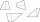Determine internal angles of isosceles trapezium ABCD /a, c are the bases/ and if: alpha:gamma = 1:3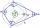What size have angles in quadrilateral (4-gon) if they are in a ratio of 8: 9: 10: 13?